# Measurement of Liquid Level

A pressure transmitter can be used to establish the liquid level in a river, tank, well, or other body of liquid. The pressure at the bottom of a liquid filled container is directly connected to the height of the liquid. The transmitter measures this hydrostatic head pressure and offers the resulting liquid level. To obtain an accurate reading, the measurement device has to be located at the lowest point one wants to measure; normally mounted or laying on the bottom of the container.

When measuring liquid level, specific gravity must be taken into consideration. Consider the following equation:

H = P/SG or P=SG • H

H – Height of the liquid being measured (usually in inches, centimeters, meters, feet, etc.)
P – Hydrostatic head pressure at the bottom of the tank (usually in inches of water column, feet of water column, bar, psi, Pascal’s, etc.)
SG – Media’s specific gravity (a dimensionless number calculated from: Density of media being measured ÷ Density of water at 4 °C. For instance, the density of kerosene equals 0.82 g/cm3. Therefore, the SG of kerosene is 0.82 g/cm3 ÷ 1.00 g/cm3 = 0.82

For instance, take a container of water 8 inches deep. Water has a specific gravity of 1.00. To calculate the hydrostatic pressure at the bottom of the container, consider:

H = 8 inches of water
SG = 1
P = x PSI
P=1 • 8 inches = 8 inches W.C.

So the hydrostatic pressure (P) at the base is equal to 8 inches of water column.

1 inch of water column = 0.03613 PSI (27.678 inches of water column = 1 PSI).
8 inches WC • 0.03613 = 0.289 PSI

So 8 inches WC = 0.289 PSI of hydrostatic pressure at the bottom of this container.

As one can see, a lower or higher specific gravity can have a significant impact on the level measurement. Other variables such as device location, temperature and/or the gas pressure acting on the media in a sealed tank are also crucial when making an accurate liquid level measurement.

The following are different recommendations for taking accurate liquid level measurements.

## Vented/Open Tanks

This is the simplest case to think about in level measurement. Vented/open tanks include elevated, inground, and above ground tanks that have open vents or expose the media to local atmospheric pressure.

Typically, the instrument can be mounted to the side of the tank (as displayed in Figure 1) or a liquid level probe can be lowered directly into the media in the tank (as shown in Figure 2). The force of hydrostatic head pressure will allow a user to obtain an accurate level reading.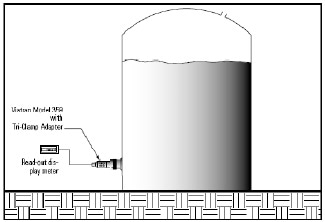Figure 1. Vented/open tank with a Tri-clamp mounted transmitter.

Let’s assume you want to measure the liquid level in a vertical tank 20 feet high. A full tank would exert a maximum hydrostatic head pressure of 20 feet of water column. (Assuming this is water at 4 °C.) Since one foot of water column is equivalent to 0.43356 PSI, the maximum hydrostatic head pressure exerted on the base of the tank and the pressure transmitter is 8.671 PSI.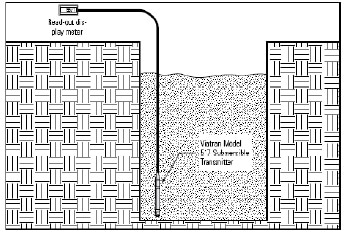Figure 2. Open tank with a submersible transmitter.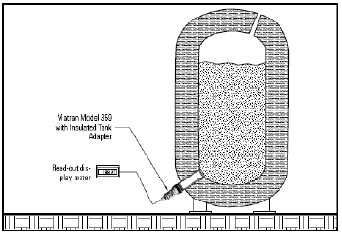Figure 3. Insulated tank with adapter.

Now, assume this same tank is full of kerosene. Since kerosene has a specific gravity of 0.82, utilizing the equation noted you get:

P = 0.82 • 20 feet = 16.4 feet water column or 16.4 feet • 0.43356 = 7.11 PSI.

In this example, there is a difference of 1.561 PSI or 3.6 feet WC between water and kerosene. This shows the significance of knowing the specific gravity of media so as to obtain an accurate level measurement.

When choosing a transmitter to measure liquid level in vented/open tanks, there are certain elementary factors that should be considered with regards to installation. Can the bottom of the tank be tapped? Can a weld-in adapter be used? What type of fitting can be inserted here? If the bottom of the tank cannot be tapped, can a level probe be inserted from the top of the tank? Is this a sanitary application where an approved product is essential or contamination issues are a concern? Is the media compatible with the standard materials or are optional wetted parts necessary?

If this is a sanitary application or the instrument can be mounted from the exterior of the tank, Viatran’s Model 359 can be used. Viatran offers many different receivers for the Model 359 to either match your current design or welding adapters for your new installation.

If this is not a sanitary application, Viatran’s Model 517 can be inserted from the top of the tank and lowered to the bottom, or Viatran’s Model 244, 344 or 544 can be plumbed via a pipe fitting to the tank base. The Model 517 is the easiest, most non-intrusive method to take a liquid level measurement. No holes or adapters are required in the tank wall and the transmitter can be easily removed without draining the tank.

If an insulated style tank is utilized, the pressure measurement is the same as stated in the above example, except a special adapter will be necessary. The adapter is welded flush on the interior of the tank and extends through the insulation to the exterior of the tank where the transmitter is inserted (see Figure 3).

## Sealed/Pressurized Tanks

A sealed tank repeatedly has a higher than atmospheric pressure gas blanket on top of the liquid. There is an additive effect of the hydrostatic head pressure (pressure exerted by the liquid level) and the pressure of the gas blanket on top. The hydrostatic pressure and gas pressure together offer a total pressure exerted at the bottom of the tank and on the level instrument.

Knowing this relationship, it is difficult to obtain an accurate liquid level measurement using the techniques as shown in the open/vented tank example. The addition of gas pressure would signify a higher liquid level, which is false.

One technique and the most accurate, is to utilize a differential pressure transducer (DP). This device will accurately measure the liquid level while negating the gas blanket pressure effect. A DP with the high side (leg) running to the base of the tank and the low side plumbed to the top of the tank above the liquid (as illustrated in Figure 4) will measure the variance between the gas pressure and the combined gas and liquid level pressure. This leaves the liquid level measurement only (barometric pressure is not a problem with this situation).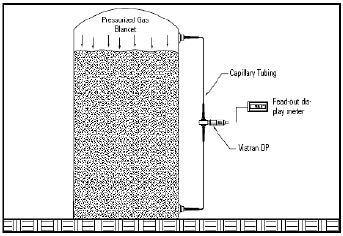Figure 4. Sealed tank with a differential pressure transducer.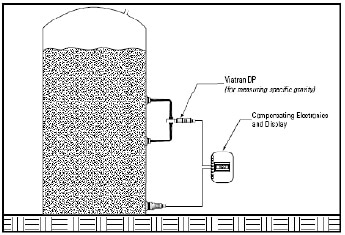Figure 5. Vented tank with changing media density.

However, there are a number of precautions and variables to consider when performing this measurement. Will the leg of each side be exposed to different temperatures? How long should each leg be? Where can the transmitter be mounted? What are the minimum and maximum temperatures of the media and gas? Is this system indoors or outdoors? Does the tank see vacuum?

To show measurement principles only, the following example will assume that optimum conditions exist. Let’s assume the tank was 30 feet high, had 20 feet of water (20 feet of water = 8.671 PSI), and has a pressurized gas blanket of 5 PSI on top. Recalling that water has a specific gravity of 1.00, the hydrostatic head pressure and gas pressure reading at the base of the tank would total 5 PSI + 20 feet WC = 13.671 PSI or 31.539 feet WC.

As illustrated in Figure 4, two remote seals are used via oil-filled capillary tubes. The low side seal of the DP is mounted above the 20 feet of water and into the gas blanket area. The high side of the DP would be mounted as close to the base as possible. The low side of the DP would sense the 5 PSI gas blanket. The transmitter subtracts the low side pressure of 5 PSI from the combined high side of 13.671 PSI or 31.539 feet WC and leaves a resulting 8.671 PSI or 20 feet WC.

Another technique, not as accurate, is to use Viatran’s Model 517 and drop this into the sealed tank. The cable end should exit the tank via a sealed feed through. A breather on the Model 517 is not essential since changes in local atmospheric pressures. A second transmitter, Viatran’s Model 570 for instance, would get mounted on top of the tank to measure just the gas blanket. The two signals would then be fed into a circuit that is capable of subtracting the two. The resultant would be the liquid level reading only. This technique is not as accurate as using a DP, but where space and installation is an issue, this will normally yield acceptable results.

## Vented or Open Tank with Changing Media Density

Some manufacturers use a single tank to process a number of media with different densities or they have media that changes density with temperature. This is very typical in the food and beverage industry where different ingredients are blended and mixed in the same tanks. An accurate liquid level measurement in these conditions utilizing a gage type instrument mounted in the base of the tank is difficult.

Utilizing a DP coupled with a gage type transmitter will provide an accurate liquid level measurement even if the density is varying. As illustrated in Figure 5, mounting the two legs of a DP a known distance apart will measure the hydrostatic head pressure between these 2 points and help you establish the specific gravity of the media. The specific gravity can then be multiplied by the level reading from the gage transmitter to acquire an accurate level reading in the system.

Let’s assume one has a tank 30 feet tall filled with 20 feet of water. As illustrated in Figure 5, if a DP is mounted on the side of this vertical tank with the low side exactly 12 inches above the high side, the output would be equivalent to 12 inches WC or 0.43356 PSI. Understanding this, you can now calculate the specific gravity of a liquid other than water.

For instance, let’s assume one has a tank with 20 feet of an unknown media. The DP is hooked up as described above, with each leg 12 inches apart. The output from the DP corresponds to a water column reading of 9.84 inches. Remember, the two legs are 12 inches apart.

Consider the following formula:

SG = DP/D

DP – Reading from the DP of the distance between DP legs (9.84 inches of water column)
D – Actual distance between DP legs (12 inches)
SG – Specific gravity

So:

SG = 9.84 inches/12 inches

SG = 0.82

Here, the specific gravity of this media is equal to 0.82.

To establish the corrected level measurement of the media at its present density, one can take the output from the gage type transmitter (Viatran’s Model 359) mounted at the base of the tank and multiply it by the specific gravity determined above. In this example, 20 feet WC is multiplied by the SG of 0.82 or 20 feet WC • 0.82 = 16.4 feet WC.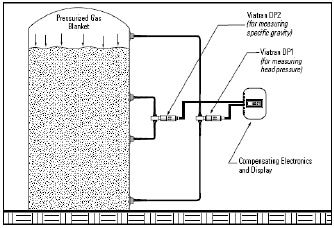Figure 6. Sealed tank with changing media density.

There are many different input devices currently available today that can take the two 4-20 mA outputs, multiply these and give a resultant 4-20 mA equivalent to the proper level.

This example shows that even with density fluctuations, one can still get an accurate liquid level measurement.

## Sealed Pressurized Tank with Changing Media Density

Here, there is a combination of both a changing specific gravity and a pressurized sealed tank. Figure 6 illustrates two DP units utilized to take an accurate liquid level measurement in this situation.

The first DP is installed like the example in a pressurized sealed tank. The low side senses the gas blanket and the high side senses the liquid level and gas blanket pressures combined (DP1). The resultant from this DP is the static pressure measurement only. However, Viatran also has a changing specific gravity.

As illustrated in the earlier example, a second DP (DP2) is mounted with its two legs 12 inches apart on the side of this vertical tank. One can then calculate the specific gravity. Once the SG is known, it is multiplied by the static pressure utilizing a math board and both DP signals. An accurate liquid level measurement can be obtained now in a pressurized tank with changing specific gravity.

These were just a few general examples of the different techniques available to solve liquid level measurement requirements.

Viatran offers a number of differential pressure transmitters that can achieve liquid level measurement accurately; Models 274, 374, 276, 376, 571, 574 or IDP10 with ranges from 0.5 inches WCD to 3000 PSID, together with remote seals, capillary tubing, and a range of fill fluids to meet your needs. Viatran also offers products such as the Model 517 that are easily set up and provide high accuracy results. For sanitary applications, the Models 350, 351, or 359 can be utilized.This information has been sourced, reviewed and adapted from materials provided by Viatran.

## Citations

• APA

Viatran. (2018, July 23). Measurement of Liquid Level. AZoM. Retrieved on December 04, 2020 from https://www.azom.com/article.aspx?ArticleID=15165.

• MLA

Viatran. "Measurement of Liquid Level". AZoM. 04 December 2020. <https://www.azom.com/article.aspx?ArticleID=15165>.

• Chicago

Viatran. "Measurement of Liquid Level". AZoM. https://www.azom.com/article.aspx?ArticleID=15165. (accessed December 04, 2020).

• Harvard

Viatran. 2018. Measurement of Liquid Level. AZoM, viewed 04 December 2020, https://www.azom.com/article.aspx?ArticleID=15165.

## Tell Us What You Think